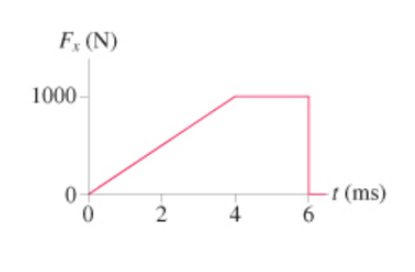# Problem: What impulse does the force shown in the figure (Figure 1) exert on a 250 g particle? Express your answer using one significant figure.

###### FREE Expert Solution

The impulse is the area under the curve. The area has two shape - A triangle and a rectangle

I = (1/2)Fmax•Δt0-4 + Fmax.Δt4-6###### Problem Details

What impulse does the force shown in the figure (Figure 1) exert on a 250 g particle? Express your answer using one significant figure.